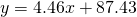# 2013 A-level H1 Mathematics (8864) Question 9 Suggested SolutionsAll solutions here are SUGGESTED. Mr. Teng will hold no liability for any errors. Comments are entirely personal opinions.

(i)

(ii)
From graphing calculator,r-value is close to 1 which suggest a strong positive correlation between the variables x and y.

(iii)
From graphing calculator, required regression line:(iv)
When.

Since r is close to 1, and we used the regression line of y on x to estimate y given an x within the range of data, the estimate will be reliable.

Not readable? Change text.